## Differentials

The derivative of a function can often be used to approximate certain function values with a surprising degree of accuracy. To do this, the concept of the differential of the independent variable and the dependent variable must be introduced.

The definition of the derivative of a function y = f(x) as you recall iswhich represents the slope of the tangent line to the curve at some point ( x, f(x)). If Δ x is very small (Δ x ≠ 0), then the slope of the tangent is approximately the same as the slope of the secant line through ( x, f(x)). That is,The differential of the independent variable x is written dx and is the same as the change in x, Δ x. That is,The differential of the dependent variable y, written dy, is defined to beThe conclusion to be drawn from the preceding discussion is that the differential of y(dy) is approximately equal to the exact change in yy), provided that the change in xx = dx) is relatively small. The smaller the change in x, the closer dy will be to Δ y, enabling you to approximate function values close to f(x) (Figure ) .Figure 1 Approximating a function with differentials.

Example 1: Find dy for y = x 3 + 5 x −1.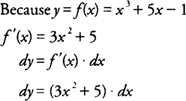Example 2: Use differentials to approximate the change in the area of a square if the length of its side increases from 6 cm to 6.23 cm.

Let x = length of the side of the square. The area may be expressed as a function of x, where y = x 2. The differential dy isBecause x is increasing from 6 to 6.23, you find that Δ x = dx = .23 cm; hence,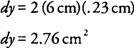The area of the square will increase by approximately 2.76 cm 2 as its side length increases from 6 to 6.23. Note that the exact increase in area (Δ y) is 2.8129 cm 2.

Example 3: Use differentials to approximate the value of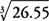to the nearest thousandth.

Because the function you are applying is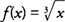, choose a convenient value of x that is a perfect cube and is relatively close to 26.55, namely x = 27. The differential dy is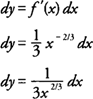Because x is decreasing from 27 to 26.55, you find that Δ x = dx = −.45; hence,which implies thatwill be approximately 1/60 less that; hence,to the nearest thousandth.

Note that the calculator value ofis 2.983239874, which rounds to the same answer to the nearest thousandth!